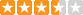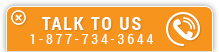# Using Excel for Soil Testing

Course Number: G-2006
Credit: 2 PDH
Subject Matter Expert: Robert Duane Nickols, P.E., R.L.S.
Price: \$59.90 Purchase using Reward Tokens. Details14 reviews
Overview

#### In Using Excel for Soil Testing, you'll learn ...

• How to calculate the moisture content of soil
• How to approximate the liquid limit of soil
• Know the order of precedence of arithmetic operators
• How to calculate the plasticity limit How to use several built-in functions in Excel- AVERAGE, STDEV, SQRT, LOG10

#### OverviewPreview a portion of this course before purchasing it.

Credit: 2 PDH

Length: 19 pages

This course covers many soils tests that can be done in Excel Liquid Limit; Plastic Limit; Sieve Analysis; Specific Gravit; Proctor.

Each test includes a description of the equipment used, the procedure used along with how the calculations and graphs look in Excel. Many engineers used to use a slide rule to calculate and graph paper to plot up the results. Excel can be used to do the calculations and plot the results. A little prior knowledge of how to use Excel is needed. This course should be of interest to Civil Engineers who might be involved with different kinds of soil testing, even if you work in the field, lab or design.

#### Specific Knowledge or Skill Obtained

This course teaches the following specific knowledge and skills:

• How to calculate the moisture content of soil
• How to approximate the liquid limit of soil
• Know the order of precedence of arithmetic operators
• How to calculate the plasticity limit How to use several built-in functions in Excel- AVERAGE, STDEV, SQRT, LOG10
• How to calculate the uniformity coefficient
• How to calculate the coefficient of curvature
• How to make an absolute cell address in Excel
• How to calculate the specific gravity of soil
• How to calculate the moist unit weight and the dry unit weight of soil

#### Certificate of Completion

You will be able to immediately print a certificate of completion after passing a multiple-choice quiz consisting of 10 questions. PDH credits are not awarded until the course is completed and quiz is passed.

Board Acceptance
 This course is applicable to professional engineers in: Alabama (P.E.) Alaska (P.E.) Arkansas (P.E.) Delaware (P.E.) Florida (P.E. Area of Practice) Georgia (P.E.) Idaho (P.E.) Illinois (P.E.) Illinois (S.E.) Indiana (P.E.) Iowa (P.E.) Kansas (P.E.) Kentucky (P.E.) Louisiana (P.E.) Maine (P.E.) Maryland (P.E.) Michigan (P.E.) Minnesota (P.E.) Mississippi (P.E.) Missouri (P.E.) Montana (P.E.) Nebraska (P.E.) Nevada (P.E.) New Hampshire (P.E.) New Jersey (P.E.) New Mexico (P.E.) New York (P.E.) North Carolina (P.E.) North Dakota (P.E.) Ohio (P.E. Self-Paced) Oklahoma (P.E.) Oregon (P.E.) Pennsylvania (P.E.) South Carolina (P.E.) South Dakota (P.E.) Tennessee (P.E.) Texas (P.E.) Utah (P.E.) Vermont (P.E.) Virginia (P.E.) West Virginia (P.E.) Wisconsin (P.E.) Wyoming (P.E.)
Reviews (14)
More DetailsPreview a portion of this course before purchasing it.

Credit: 2 PDH

Length: 19 pages